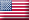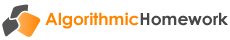# Mathematical Techniques in Finance Edition 2Thoroughly explores the advanced mathematical techniques used in modern finance. Requiring comprehensive knowledge of the underlying principles and utilising methods from calculus, this e-workbook facilitates mastery of the theory of interest and stimulates rigorous analysis of the techniques used in pricing, financing, investing, trading and managing risks and returns. The e-workbook combines academic thought, proof, theory and the application of formulas.

#### Measuring interest involved in single payment transactions

• Interest accumulation
• Nominal and effective rates of interest and discount
• The force of interest
• Future value at simple, compound and continuous rates
• Present value at simple, compound and continuous rates
• Equations of value

#### Annuity valuation

• Future value of an ordinary annuity
• Future value of an annuity-due
• Present value of an ordinary annuity and an annuity-due
• Solving for the term, payment and yield of an annuity
• Valuation of deferred annuities
• Present value of a perpetuity as the limit of an annuity
• Valuing annuities with varying interest and periodic payments
• Continuous annuities
• Valuing annuities with inconsistent compounding and payment frequencies
• Valuing annuities that form a geometric progression
• Valuing annuities that form an arithmetic progression
• Applications to reinvestment risk, depreciation and other real world problems

#### Loans

• Amortisation method of loan repayment
• Developing an amortisation schedule
• Retrospective and prospective methods of loan outstanding
• Adjusting the repayment and/or term in response to changes in the loan interest rate
• Sinking-fund method of loan repayment
• Applications to valuing loans and other real world problems

#### Valuation of bonds

• Pricing a bond on a coupon date
• Pricing a bond between coupon dates
• Solving for the yield to maturity implied in a bond purchase
• Holding period return allowing for reinvestment rates
• Amortisation of a bond
• Allowing for income and capital gains tax on bonds
• Applications to callable and serial bonds

#### Probability and random variables

• Introduction to the theory of probability
• Rules of probability and enumeration
• Random variables
• Probability distributions
• Expected value and variance
• Applications to portfolio theory

#### The rate of return on an investment

• Internal rate of return and net present value
• Payback period
• Profitability index
• Dollar-weighted rate of return
• Time-weighted rate of return
• Applications to investment decisions and fund management with continuous transactions

#### The term structure of interest rates

• Spot and forward rates
• Yield curves
• The relationship between spot rates and bond yields
• Applications to arbitrage and interest rate swaps
• Duration and immunisation
• Duration of a series of cash flows and bond duration
• Convexity of a series of cash flows
• Asset-liability matching and immunisation
• Applications to interest rate risk management

#### Valuing securities and financial instruments

• Pricing using the arbitrage principle
• Forward and futures contracts
• Modelling shares using the CAPM approach
• Modelling using the binomial and Black-Scholes approaches
• Fixed income investments and bond default risk
• Foreign exchange rates and the interest rate parity theorem